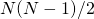# Hierarchical Risk Parity (HRP)

Hierarchical risk parity (HRP) is a portfolio optimization approach that does not require inversion of the covariance matrix. HRP is a more robust way of constructing portfolios. Hierarchical risk parity was first suggested by Marcos Lopez De Prado in 2016.

On this page, we discuss the main steps of the hierarchical risk parity approach and discuss why it is more robust that traditional mean-variance optimization. Generally, it is not possible to perform HRP using a simple Excel spreadsheet. Instead, investors should use statistical software such as R, Python, or MATLAB.

## Weaknesses mean-variance optimization

To perform a traditional mean-variance optimization, as suggested by Harry Markowitz in 1952, we need to use a covariance matrix. The difficulty is not the covariance matrix itself, but rather that the covariance matrix is hard to estimate in practice. This is because we need a large number of observations to estimate a covariance matrix. To give an example, suppose we have 50 assets (N). In that case, the covariance matrix is 50 x 50. To estimate all the off-diagonal elements, we need to estimateparameters.

In this case, we need to estimate a total of 1225 parameters. Generally, if the number of observations T is lower than N, the covariance cannot be estimated. Worse still, it is not possible to take the inverse. And it is exactly this inverse that is needed to perform a traditional mean-variance optimization.

## Hierarchical risk parity

To solve the problem, hierarchical risk parity takes another approach. Instead of inverting the covariance matrix, the matrix is analyzed. In particular, hierarchical risk parity applies hierarchical clustering. This means that the securities in the universe are clustered together. After clustering the securities, the covariance matrix is then reordered, such that similar stocks are close to each other. Finally, the portfolio is optimized by performing and inverse volatility weighting approach where risk is allocated to the clusters iteratively.

## Summary

We discussed hierarchical risk parity, a new version of risk parity that does not require investors to invert the covariance matrix. This new approach leads to more robust portfolios that perform well out-of-sample.